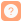题目内容 （请给出正确答案）
[主观]

# 设x为整型变量,p是指向整型的指针变量,则下列赋值表达式正确的是（)。A.p=&xB.p=xC.*p=&xD.*p=*x请帮忙给出正确答案和分析，谢谢！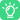答案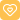更多“设x为整型变量,p是指向整型的指针变量,则下列赋值表达式正确的是（)。A.p=&xB.p=xC.*p=&xD.*p=*x请帮忙给出正确答案和……”相关的问题

A.M个指向整型变量的指针
B.指向M个整型变量的函数指针
C.一个指向具有M个整型元素的一维数组的指针
D.具有M个指针元素的一维指针数组，每个元素都只能指向整型量

A.指向字符型的指针
B.字符型
C.整型
D.指向整型的指针

A.整型常量
B.字符型常量
C.数组名
D.整型表达式

Dim a1,a2 as integer 语句声明变量a1和a2都为整型变量。（）

A.b=a/2
B.b=a%2
C.b=8-(3,a-5)
D.b=a>5？4:2

A.4
B.false
C.3
D.true

A.k=*p1+*p2
B.k=*p1*(*p2)
C.p2=k
D.p1=p2下载APP关注公众号
TOP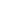•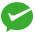微信支付
•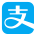支付宝支付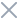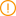重要提示：请勿将账号共享给其他人使用，违者账号将被封禁。